# Noise Figure of Passives

This material was contributed by The Professor back in November 2010, around the time he passed his 50th trip around the nearest star. Thanks!"Just like at your company, on Gilligan's Island we
always had a shortage of people that knew technology!"

Before we get all technical, it must be reported that Sherwood Schwartz, born in 1916 in Passaic New Jersey (separated by enough distance from where the Unknown Editor was born so there's room for both of their statues), producer of Gilligan's Island, received his Hollywood Walk of Fame Star in 2008. Rumor has it that Schwartz is planning a GI remake to be shown in 2011. Best wishes and Happy Chanukah from Microwaves101.com!

New for June 2013! We have some further comments from Matt on this topic which appear below. Thanks!

A) Say you're putting 1 mW of power incident into port 2 of a lossy, passive part. That 1 mW gets scattered three ways. Some of it is reflected, some is transmitted through, and some is dissipated as heat. The part that's dissipated is: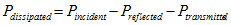Normalizing this to the incident power gives: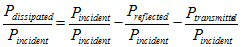In terms of S-parameters, that comes out as: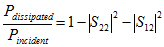Generation of thermal noise is like a time-reversal of power dissipation. Instead of electrical energy being converted to heat, heat is converted to electrical energy in the form of noise. Since we're concerned with the noise power that comes out of port 2 for this analysis, the "|S12|2" term above needs to be replaced with |S21|2. This results in a thermally-generated noise density term of: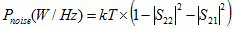Where k is Boltzmann's constant and T is the component's absolute temperature in Kelvins.

A little clarification is in order... specifically, why did the |S12|2 in the first equation become |S21|2 in the second? It's because those "i" and "j" subscripts in "Sij" denote receive and transmit ports, respectively. Since we're treating reception as the time reversal of transmission, the port that was transmitting in the first equation became the port that's receiving in the second equation, and vice-versa. So, |S12| becomes |S21| when you reverse the direction of time. Technically, the "i" and "j" in the |S22|2 term get swapped too, but since they're both 2 it doesn't show up.

B) The total noise power that comes out of a component contains two terms. The first is the noise power incident on the input of the component that gets through to the output, and the second is the internally-generated noise. In the definition of noise figure, the input noise power density is always k * To, or -173.98 dBm/Hz. So the total output noise power density of a lossy, passive component is: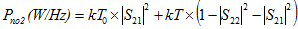(1)

Where To = 290 Kelvin but T = the part's temperature in Kelvin. This gives you the noise power density coming out of port 2. There's a corresponding equation for the noise density coming out of port 1 that looks like: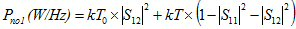C) This just gives you the output noise power density of a lossy part. That still has to be converted to noise figure. The noise power density at the output of any noisy (or noiseless) part in a system is: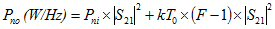(2)

Where Pni is the input noise power density and F is the noise figure (not in dB). If the input noise power density Pni is k * To = -173.98 dBm/Hz, the output noise power density simplifies to the familiar expression: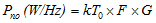(where G = |S21|2), or in dB: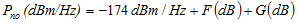Equating (1) and (2) gives: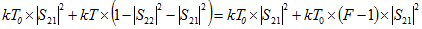Subtracting the k * To * |S21|2 terms from both sides and canceling the k terms and rearranging gives: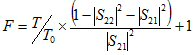This can also be expressed as: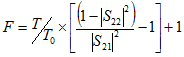(3)

So, if the part temperature is equal to To (290 K), and |S22| = 0, the noise figure is equal to the reciprocal of the gain, i.e., the noise figure is equal to the loss. If the part is colder than 290 K, the noise figure is less than the loss, and conversely if the part is hot the noise figure will be higher. Also, the noise figure is reduced by the reflections, or more accurately, by the part of the loss that comes from reflections. A simple way to say this is that the noise figure of a lossy part is based on the dissipative part of the loss, and the part's absolute temperature.

Contributed June 2013 (thanks!)

I believe the final expression should be F=1+T/T0*(1/Gav-1), which simplifies down to the expression given on that page if you take the simplification that GammaS=GammaL=0. This is a good approximation as long as things are pretty well matched but it's not the case when doing something like measuring noise parameters with a loadpull system or measuring unmatched transistors.

We know from the definition of noise factor that F for a passive network at T0 is simply 1/Gav so any expression for F of a passive must simply down to this for a temperature T=T0.

I have a slightly different derivation than the Professor's which makes more intuitive sense to me. But I think that the Professor's derivation is correct and the simplification in the result come from using S22 and S12 for reflected and transmitted power which is only 100% correct when GammaS=GammaL=0.

Author : The Professor